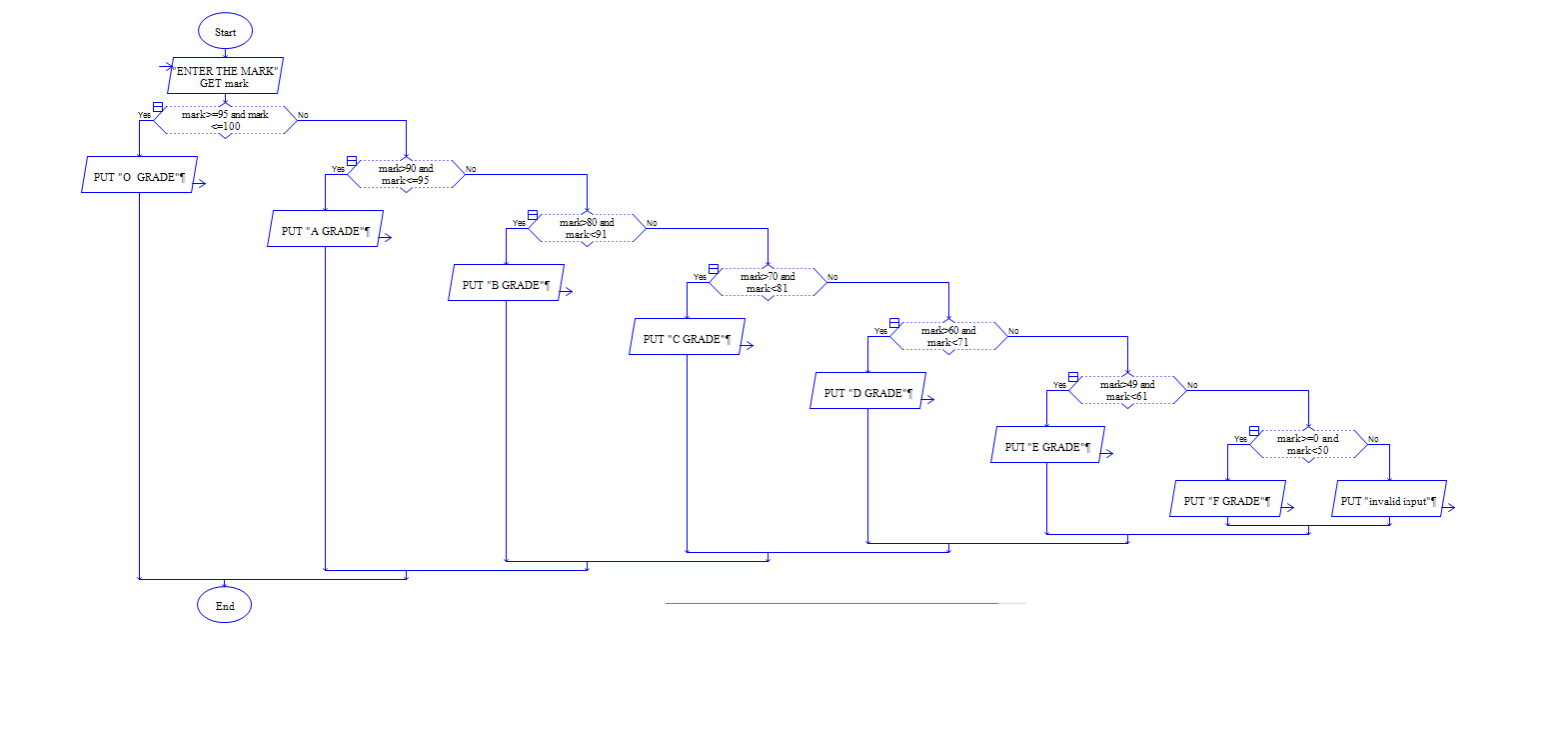Python Program to get a mark of a student and find its grade.

Sample Input 1:

75

Sample Output 1:

C

#### Flow Chart Design#### Program or Solution

``` mark=int(input("Enter Mark:")) if(mark>95 and mark<=100): print("O Grade") elif(mark>90 and mark<=95): print("A grade") elif(mark>80 and mark<91): print("B Grade") elif(mark>70 and mark<81): print("C Grade") elif(mark>60 and mark<71): print("D Grade") elif(mark>49 and mark<61): print("E Grade") elif(mark>=0 and mark<50): print("F Grade") else: print("Invalid Mark") ```

#### Program Explanation

chained conditional check is used here to check the mark range.

any one of the grade will be displayed.